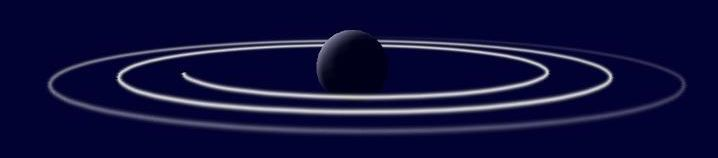## BEAM

The particle is "smeared" along a line given by its velocity vector and starting at its position. Normally used for continuous rays or trails. In combination with the BRIGHT flag, lines of light can be created this way for laser, tracer bullet, vapor or light trail effects.

## STREAK

Like BEAM, but stretches the particle along its velocity vector, instead of smearing it. This gives the effect a motion blur like appearance.

### Type:

flag, particles only

C

### Remarks:

• When one of these flags are set, instancing can not be used for accelerating particle rendering.
• A8.4 Through particle_mode a 2D or 3D mode can be selected for BEAM and STREAK. In 2D mode, beams are generated by drawing many particles over each other, resulting in a slightly different looking (and slower) effect. 2D mode is not supported in isometric views.
• For vapor trails, use the BEAM flag and set the velocity vector to the difference of the current emitter position to its position in the last frame.

### Example (lite-C):```function p_alphafade(PARTICLE *p)
{
p.alpha -= p.skill_a*time_step;
if (p.alpha <= 0) p.lifespan = 0;
}

function p_trace(PARTICLE *p)
{
set(p, BRIGHT|TRANSLUCENT|BEAM);
p.size = 2;
p.skill_a = 1; // fade factor
}

// entity that runs in circles and leaves a vapor trail
action tracer()
{
var dist = 0,radius = 10,sign = 1;
VECTOR last_pos;
while(1)
{
vec_set(last_pos,my.x);
dist += 30*time_step;
if (radius > 150) sign = -1;
else if (radius < 30) sign = 1;
effect(p_trace,1,my.x,vec_sub(last_pos,my.x));
wait(1);
}
}

function main()
{
vec_set(sky_color,vector(50,1,1)); // dark blue